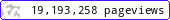•••••MadAsMaths.com :: Maths Booklets :: Basic Topics :: Various

BASIC TOPICS - VARIOUS

These booklets are suitable for

• providing enrichment material for very able G.C.S.E students. (most booklets)

• covering the typical first year non calculus material, of a two year course in A Level mathematics.

• introducing/revising various non calculus topics to undergraduate students in degrees not requiring A Level mathematics.

 3d_geometric_mensuration.pdfalgebraic_fractions.pdfalgebraic_fractions_exam_questions.pdfarithmetic_series_exam_questions.pdfarithmetic_series_worded_questions.pdfbinomial_expansions_exam_questions.pdfbinomial_expansions_practice.pdfcircle_coordinate_geometry_exam_questions.pdfcubic_sketching.pdfcubics_exam_questions.pdfdiscriminant_exam_questions.pdfdiscriminant_practice.pdfequations_exam_questions_i.pdfgeometric_mensuration.pdfgeometric_series.pdfgeometric_series_worded_questions.pdfidentities.pdfindices_exam_questions.pdfindices_practice.pdfindices_practice_student_version.pdfinequalities_basic_practice.pdfinequalities_exam_questions.pdfline_coordinate_geometry_exam_questions.pdfline_coordinate_geometry_practice.pdflogarithms_exam_questions.pdflogarithms_practice.pdfpolynomials_exam_questions_intro.pdfpolynomials_practice_intro.pdfquadratic_sketching.pdfquadratics_exam_questions.pdfreciprocal_functions.pdfrecurrence_relations.pdfseries_mixed_exam_questions.pdfsurd_exam_questions.pdfsurds.pdfsurds_student_version.pdfvector_algebra_introduction.pdf